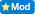4.5% of 170 is what number

0  Views: 504 Answers: 6 Posted: 11 years ago

Let us see the solution for your problem:

Problem: 4.5% of 170

= 4.5 / 100 × 170

[ Tips- always convert the given percentage into simple whole number fraction]

= 45/1000 × 170

[Tips - Now simply the remaining expression]

[ Use the simple division rule or you can use your calculator for fast result]

= 45/100 × 17

= 9/ 20 × 17

= 153/20

= 7.65

So the correct answer is 7.65.

Hope this has helped you to some extent. Now solve the other related problems with your friends.

DuckyAhhh...memories of my high school math class.

170 x .045 = 7.65    OR
1% = 1.7     (170/100)    1/2% = .86  (1.7/2)
(1.7 x 4) + .86 = 7.65
6.8  + .86 = 7.65

Poppy3

All too brilliant you and the others.

Good homework everyone.I have decided to start marking all of these homework questions.

"A+"

All of you CRACK me up!! I love it!

ROMOS
Karma: 2940

Bob/PKB
Karma: 1830

Colleen
Karma: 1760

Benthere